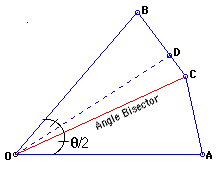# Approximate with Technology: Trisecting AngleIt is a well-known fact that trisecting an angle with Greek construction rules is impossible. But this does not mean that one can not provide a construction, which approximates the trisection. Use a dynamic geometry software and a spreadsheet determine how well is the construction described below. Construction: Given an angle AOB, construct the bisection AOC, with OA = OC = OB. Then construct a trisection of BC such that BD = 2CD. Then the angle DOB is approximately the trisection of the angle AOB. Is it? Explain why or why not.Extension: